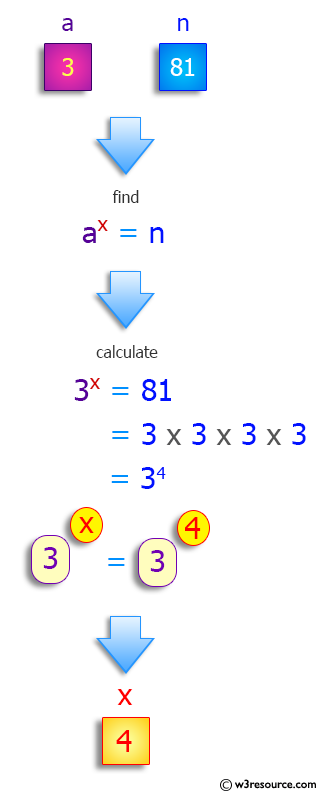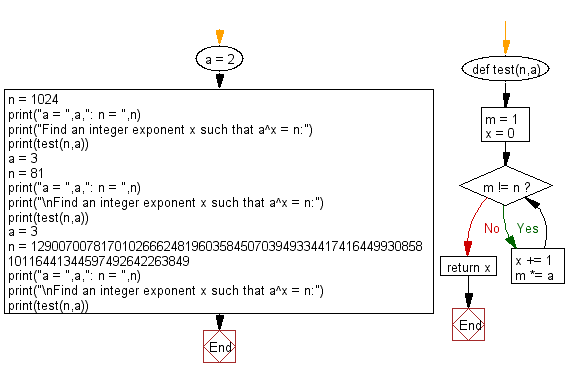﻿ Python: Find an integer exponent x such that a^x = n - w3resource# Python: Find an integer exponent x such that a^x = n

## Python Programming Puzzles: Exercise-56 with Solution

Write a Python program to find an integer exponent x such that a^x = n.

```Input:
a =  2 : n =  1024
Output:
10
Input:
a =  3 : n =  81

Output:
4
Input:
a =  3 : n =  1290070078170102666248196035845070394933441741644993085810116441344597492642263849

Output:
170
```

Pictorial Presentation:Sample Solution:

Python Code:

``````def test(n,a):
m = 1
x = 0
while m != n:
x += 1
m *= a
return x

a = 2
n = 1024
print("a = ",a,": n = ",n)
print("Find an integer exponent x such that a^x = n:")
print(test(n,a))
a = 3
n = 81
print("a = ",a,": n = ",n)
print("\nFind an integer exponent x such that a^x = n:")
print(test(n,a))
a = 3
n = 1290070078170102666248196035845070394933441741644993085810116441344597492642263849
print("a = ",a,": n = ",n)
print("\nFind an integer exponent x such that a^x = n:")
print(test(n,a))
``````

Sample Output:

```a =  2 : n =  1024
Find an integer exponent x such that a^x = n:
10
a =  3 : n =  81

Find an integer exponent x such that a^x = n:
4
a =  3 : n =  1290070078170102666248196035845070394933441741644993085810116441344597492642263849

Find an integer exponent x such that a^x = n:
170
```

Flowchart:## Visualize Python code execution:

The following tool visualize what the computer is doing step-by-step as it executes the said program:

Python Code Editor :

Have another way to solve this solution? Contribute your code (and comments) through Disqus.

What is the difficulty level of this exercise?

Test your Programming skills with w3resource's quiz.

﻿

## Python: Tips of the Day

Clamps num within the inclusive range specified by the boundary values x and y:

Example:

```def tips_clamp_num(num,x,y):
return max(min(num, max(x, y)), min(x, y))
print(tips_clamp_num(2, 4, 6))
print(tips_clamp_num(1, -1, -6))
```

Output:

```4
-1
```